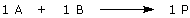# Molar Equivalents

Some laboratory instructions express the amount of reagent that is needed as “XXX molar equivalents.” This instruction is defining the amount of reagent to be used relative to another compound used in the experiment. Therefore, to interpret these instructions correctly, you must pay attention to their context.

An example: Assume that you had previously obtained 12 g of compound A from an earlier experiment, and now you want to combine A with compound B to make compound P. Let’s assume too that a catalyst, compound Z, is required for this reaction to occur and the reaction stoichiometry is given by:The instructions for this experiment might read: “Combine A from the previous experiment with B (1.1 molar equivalents) and Z (0.01 molar equivalents).”

You can interpret these instructions as follows:

1. Use all 12 g of A obtained from the previous experiment
2. Calculate the number of moles of A contained in 12 g of A. Call this “MA”
3. Use 1.1(MA) moles of B
4. Use 0.01(MA) moles of Z

In other words, the instruction “molar equivalents” (or sometimes just “equivalents”) refers to the amount of compound A measured in moles. The instructions are intended to guarantee that the compounds are used in the following molar ratio:

A : B : Z = 1 : 1.1 : 0.01